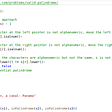# Leetcode — 560. Subarray Sum Equals K

The link to the problem: https://leetcode.com/problems/subarray-sum-equals-k/

Given an array of integers `nums` and an integer `k`, return the total number of continuous subarrays whose sum equals to `k`.

Constraints:

• `1 <= nums.length <= 2 * 104`
• `-1000 <= nums[i] <= 1000`
• `-107 <= k <= 107`
`ExampleInput: nums = [1,2,3], k = 3Output: 2 since both continuous subarrays [1,2] and  have the sum of 3`

# Thoughts:

My first thought is to use brute force to solve the problem. We try every possible subarray that starts at index i and will see if we can reach the target sum of k. This will require O(n²) time complexity since we will try every combination of all the subarrays.

Below is the 1211ms code that beats 20% —

`public int subarraySum(int[] nums, int k) {        int count = 0;        int n = nums.length;        for(int i=0;i<n;i++)        {            int temp = 0;            for(int j=i;j<n;j++)            {                temp += nums[j];                if(temp==k)                    count++;            }        }        return count;    }`

Although we can successfully pass all the cases, it seems like we can use some extra space to reduce the time complexity.

Can we try to use O(n) time to solve the problem? Let’s draw down the process of how we find the subarray sums and see if there’s any pattern.

## 👷For example, if we have an input array, nums= [1, 2, 3, 4, 1].

The below graph shows the process of the above code.

⚠️To make everything easier to read, I will use nums[i, j] to represent the subarray we formed from index i through index j. Hence in this example, nums[0, 2] represents the subarray [1,2,3].

We write down each sum of all the possible subarray nums[i, j]. Hence, sums in the same row mean these subarrays have the same starting position.
(These numbers in orange are exactly the values that we use the “temp” variable to calculate in the above code.)

If you look at the graph carefully, you can see that except for the first row (the sums of the subarrays that start at index 0), we can get all other sums by using the sums of nums[0, i] subtracted by nums[0, j] where 0, i, j are the indices in the array and 0≤j<i. (The “new sum” we get from the sum of nums[0, i]- the sum of nums[0, j] represents the sum of nums[j+1, i]. )

For example, if we take a look at all the sums end at index 3. You can see that we can get 9, 7, and 4 by using the sum of the subarray [1,2,3,4] subtracted by the sums of the subarray , [1,2], or [1,2,3]. That is to say, we will actually get the sums of the subarray like [2,3,4], [3,4], and .

# Code Logic:

Hence, we can do some modification to the above code. We still need the temp variable to help us count the sums of nums[0, i] where i can be any position in the array. This time, we need an extra variable to help us record the sums we have met. (i.e., this variable should contain the sums of nums[0, j] where j is from 0 through i-1)

I decided to use a Set to stores all the sums we have met since the time complexity of checking if a number contains in a set is O(1) in Java. We need to run a loop to traverse every position in the nums array, and there are three things we need to do in each iteration when the current position is i.

1. Add the current position number nums[i] to the temp variable. (so the temp will always contain the sum of nums[0, i])
2. Check if we can get the target sum k by subtracting any sums of nums[0, j] where j<i from the sum of nums[0, i]
3. Store the current sum (i.e., nums[0, i]) into the set

Also, we need to add 0 into the set before we start the iteration since it is possible that nums[0, i] already reach the target sum k.

Then I came up with this code:

`public int subarraySum(int[] nums, int k) {        int count = 0;        int n = nums.length;        Set<Integer> sums = new HashSet<>();        sums.add(0);        int temp = 0;        for(int i=0;i<n;i++)        {            temp+= nums[i];            if(sums.contains(temp-k))                count++;            sums.add(temp);        }        return count;    }`

This code will fail at the test case [-1,1,0,0], k = 0. The reason is pretty obvious since I forgot to take care of the possibility that nums[0, j] and nums[0, j’] might have the same sums while j != j’. Hence, we can’t simply use Set to store all the previous sums. We need to use a Map to store each sum and the number of times it appears.

Hence, we can use the below 19ms code to pass all the test cases using O(n) time complexity

`public int subarraySum(int[] nums, int k) {        int count = 0;        int n = nums.length;        Map<Integer,Integer> map = new HashMap<>();//sum - count        map.put(0, 1);        int temp = 0;        for(int i=0;i<n;i++)        {            temp+= nums[i];            count += map.getOrDefault(temp-k,0);            map.put(temp, map.getOrDefault(temp,0)+1);        }        return count;    }`

--

--

--

## More from Anj

Click here to view all the stories posted on this blog: https://anj910.medium.com/bookmark-c95e4c52cf44

Love podcasts or audiobooks? Learn on the go with our new app.

## 如何自動佈署隱藏式Kali虛擬機## Best practices for test automation## Introduction to Ansible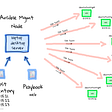## Step-by-Step on how to display currency symbols like ₦ or Kč in flutter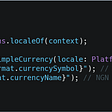## Building Server-Sent Event (SSE) API using Go and Redis Keyspace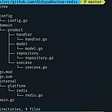## Incompatibility of Conventional Database with Patent Search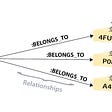## Anj

Click here to view all the stories posted on this blog: https://anj910.medium.com/bookmark-c95e4c52cf44

## Deleting a Node from Binary Search Tree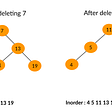## Finding the relationship between different sorting algorithms with respect to size of integer data…## 125. Valid Palindrome